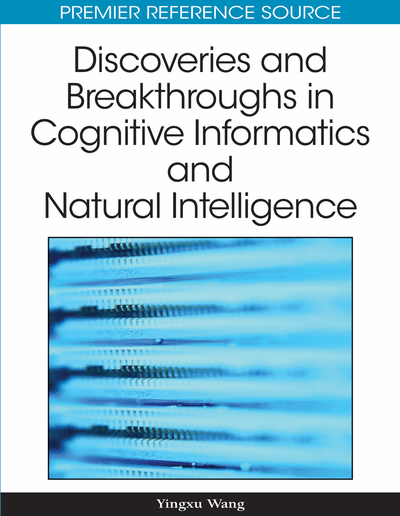# On the Big-R Notation for Describing Iterative and Recursive Behaviors

Yingxu Wang (University of Calgary, Canada)
DOI: 10.4018/978-1-60566-902-1.ch015
Available
\$33.75
List Price: \$37.50
10% Discount:-\$3.75
TOTAL SAVINGS: \$3.75

## Abstract

Iterative and recursive control structures are the most fundamental mechanisms of computing that make programming more effective and expressive. However, these constructs are perhaps the most diverse and confusable instructions in programming languages at both syntactic and semantic levels. This article introduces the big-R notation that provides a unifying mathematical treatment of iterations and recursions in computing. Mathematical models of iterations and recursions are developed using logical inductions. Based on the mathematical model of the big-R notation, fundamental properties of iterative and recursive behaviors of software are comparatively analyzed. The big-R notation has been adopted and implemented in Real-Time Process Algebra (RTPA) and its supporting tools. Case studies demonstrate that a convenient notation may dramatically reduce the difficulty and complexity in expressing a frequently used and highly recurring concept and notion in computing and software engineering.
Chapter Preview
Top

## The Big-R Notation

Although modern high-level programming languages provide a variety of iterative constructs, the mechanisms of iteration may be expressed by the use of conditional or unconditional jumps with a body of linear code. The proliferation of various loop constructs in programming indicates a fundamental need for expressing the notion of repetitive, cyclic, recursive behaviors, and architectures in computing.

In the development of RTPA (Wang, 2002, 2003, 2006a, 2007a, 2007b), it is recognized that all iterative and recursive operations in programming can be unified on the basis of a big-R notation (Wang, 2006b). This section introduces the big-R notation and its mathematical foundation. It can be seen that a convenient notation may dramatically reduce the difficulty and complexity in expressing a frequently used and highly recurring concept and notion in programming.

## Complete Chapter List

Search this Book:
Reset